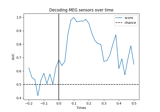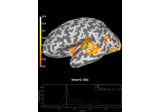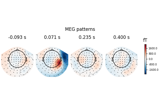# mne.decoding.get_coef#

mne.decoding.get_coef(estimator, attr='filters_', inverse_transform=False)[source]#

Retrieve the coefficients of an estimator ending with a Linear Model.

This is typically useful to retrieve “spatial filters” or “spatial patterns” of decoding models .

Parameters:
estimatorobject | `None`

An estimator from scikit-learn.

attr`str`

The name of the coefficient attribute to retrieve, typically `'filters_'` (default) or `'patterns_'`.

inverse_transform`bool`

If True, returns the coefficients after inverse transforming them with the transformer steps of the estimator.

Returns:
coef`array`

The coefficients.

References

## Examples using `mne.decoding.get_coef`#Decoding (MVPA)

Decoding (MVPA)Decoding source space data

Decoding source space dataLinear classifier on sensor data with plot patterns and filters

Linear classifier on sensor data with plot patterns and filters